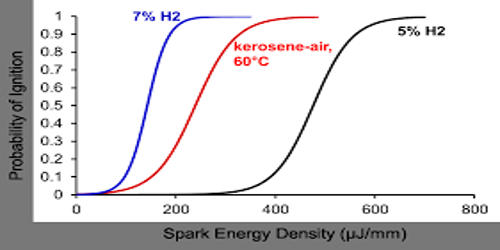Chemistry

# Vapour Pressure of KeroseneThe vapor pressure of a liquid is defined as the pressure exerted by the molecules that escape from the liquid to form a separate vapor phase above the liquid surface. This pressure is formed in a thermodynamic equilibrium state in a closed container at a certain temperature. Liquid’s evaporation rate is identified by the equilibrium vapor pressure.

Kerosene is a flammable liquid mixture of chemicals that are produced in the distillation of crude oil. To produce kerosene, crude oil is distilled in a distillation tower in a process similar to that used to produce diesel and gasoline. RVP values for kerosenes are much lower than for gasoline as would be expected from their higher boiling range. An equation of the form:

P = Aexp(-B/T)

where P is the vapor pressure, T the temperature and A and B are constants has sometimes been applied to the vapor pressure of kerosenes. Such an equation applies rigorously only to pure hydrocarbon compounds, being an integrated form of the Clausius-Clapeyron equation. In this case, B is the heat of vaporization divided by the gas constant. That complex mixtures of hydrocarbons when examined experimentally often conform to such a functional form is well known. In reference, several kerosene jet fuels were examined for the temperature dependence of vapor pressure, and the results fitted to an equation of the form above. Kerosene tends to contain hydrocarbons that have anywhere from 11 to 13 carbons in the chains. Liquid kerosene fuels contain potentially harmful compounds, including hexane and benzene. One of the fuels was found to fit the equation:

P/kPa = 6905exp(-2196.53/T)

Putting T = 311K which is the temperature of an RVP measurement: P= 5.9 kPa

It is a medium weight distillate in the refining process and can be produced by distilling crude oil or by hydrocarbon cracking heavier petroleum. A similar fuel was found to conform to: P/kPa = 2779.43exp(-1773.77/T) giving a RVP of 9.3 kPa. Work cited in reference gives, for another kerosene jet fuel:

P/kPa = 653436.08exp(-4243.3/T) giving an RVP of 0.78 kPa (6 mm Hg). That some kerosenes do have RVP values as low as this is well known.

When with results of the type outlined above ln(P/kPa) is plotted against 103K/T the result is a line of slope -B (units K). As we have seen, for a pure compound:

B = ΔHvap/R

So a steep slope would signify a high heat of vaporization. We expect intuitively that the same would apply to the kerosenes. In the example equations given above the constant in the exponential varies by a factor in excess of two. That the one with the highest value of B is the one with the lowest RVP is significant. The chemical composition of kerosene is fairly complex, and it is a complex mixture of paraffin (55.2%), naphthenes (40.9%), and aromatic hydrocarbons (3.9%).